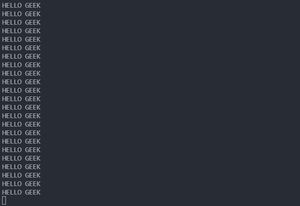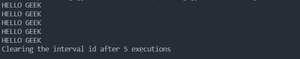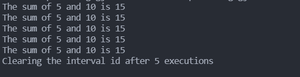# What is the use of setInterval() method in Node.js ?

• Last Updated : 30 Sep, 2021

The setInterval() method helps us to repeatedly execute a function after a fixed delay. It returns a unique interval ID which can later be used by the clearInterval() method which stops further repeated execution of the function.

Syntax:

```const intervalId = setInterval(func,
[delay, arg1, agr2, ..., argN]);```

where,

• func is the function that we want to execute repeatedly after delay milliseconds.
• delay (optional parameter) is the number of milliseconds delay between two repeated execution of the function.
• arg1, …, argN (optional parameter) are the arguments that will be passed to func when it is executed.

Example 1: In this example, we will see Infinite number of function executions.

When we use the setInterval() method and do not clear it using the clearInterval() method then it keeps on executing the function passed to it a limitless number of times. We can use the setInterval() method like this when we need to fetch some information after every fixed interval like when we are building a clock and we need to calculate and update the number of seconds after every second. A simple code example demonstrating this type of use of setInterval() method is mentioned below.

## Index.js

 `setInterval(() => { ` `  ``console.log(``'HELLO GEEK'``); ` `}, 1000); `

Output:Example 2: In this example, we will see Finite number of executions using clearInterval(). If we want the function to execute a limited number of times then we can use clearInterval() method. We can clear an interval by passing the interval id that is returned from the setInterval() method to clearInterval() method.

Syntax:

`clearInterval(intervalId)`

The code example mentioned below demonstrates the use of clearInterval() method to attain finite repeated executions of the function.

## Index.js

 `let count = 0; ` ` `  `const intervalId = setInterval(() => { ` `  ``console.log(``'HELLO GEEK'``); ` `  ``count++; ` ` `  `  ``if` `(count === 5) { ` `    ``console.log(``'Clearing the interval id after 5 executions'``); ` `    ``clearInterval(intervalId); ` `  ``} ` `}, 1000); `

Output:Example 3: In this example, we will see Using the arguments passed to setInterval(). The arguments that are passed to the setInterval() method after the delay parameter are received by our function and can be used in the desired manner. The code mentioned below demonstrates it with the help of a simple code example.

## Index.js

 `let count = 0; ` ` `  `// The arguments passed after the  ` `// delay (in milliseconds) will ` `// be received in our function  ` `// inside the setInterval() method ` `const intervalId = setInterval( ` `  ``(a, b) => { ` `    ``console.log(`The sum of \${a} and \${b} is \${a + b}`); ` `    ``count++; ` ` `  `    ``if` `(count === 5) { ` `      ``console.log(``"Clearing the interval id after 5 executions"``); ` `      ``clearInterval(intervalId); ` `    ``} ` `  ``}, ` `  ``1000, ` `  ``5, ` `  ``10 ` `); `

Output:My Personal Notes arrow_drop_up
Recommended Articles
Page :